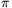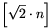Mathematical and Physical Journal
for High Schools
Issued by the MATFUND Foundation
 Already signed up? New to KöMaL?

# New exercises and problems in MathematicsJanuary 2002## New exercises in January 2002

Maximum score for each exercise (sign "C") is 5 points.

C. 655. Whenever there is a lottery draw, Barnie's grandmother sets aside some change in a piggy bank for her grandson. Grandma is a lady of firm discipline; she always observes the following rules:

1) She only puts coins in the piggy bank.

2) When a number is drawn, she immediately places that number of forints (HUF) in the piggy, using the lowest possible number of coins.

3) When the lottery draw is over, she counts how many of each kind of coin she has put in the piggy.

One day, when 7 numbers were drawn out of the first 35 positive integers, she counted 3 20-forint coins, 6 10-forint coins, 5 5-forint coins, 9 2-forint coins, and 3 1-forint coins. What were the 7 numbers drawn?

Note: There exist the following coins in Hungarian currency: 100, 50, 20, 10, 5, 2, and 1-forint (HUF) coins.

C. 656. A jacket with an original price of 21,250 forints (HUF) was put on sale. Then, with the start of the great Christmas sale, its price was reduced again, this time to 19,176 forints. By what percentage was the price reduced each time, given that both percentages of reduction were one-digit numbers?

C. 657. A cone and a cylinder have equal heights and equal volumes. Find the apex angle of the cone if the areas of the lateral surfaces are also equal!

C. 658. Solve the simultaneous equations

$\displaystyle {1\over x}+{1\over y}={1\over z}$

$\displaystyle {1\over x+y}+{1\over y-6}={1\over z}$

$\displaystyle {1\over x+24}+{1\over y-15}={1\over z}.$

C. 659. For what values of the real parameter 0$\displaystyle le$tdoes the equation sin (x+t)=1-sinx have no solution?## New problems in January 2002

The maximum scores for problems (sign "B") depend on the difficulty. It is allowed to send solutions for any number of problems, but your score will be computed from the 6 largest score in each month.

B. 3512. Find the lowest multiple of 81 in which a) all digits are ones. b) all digits are ones and zeros. (4 points)

B. 3513. Prove that if two circles are not coplanar and they have exactly two common points, then there exists a sphere that contains both circles on its surface. (3 points)

B. 3514. The altitudes of triangle ABC are AA1, BB1 and CC1, its area is a. Prove that the triangle ABC is equilateral if and only if AA1.AB+BB1.BC+CC1.CA=6a. (3 points)

B. 3515. Prove that there exist infinitely many powers of 2 among the numbers of the form. (4 points)

B. 3516. We have drawn a closed polygon of length 5 cm on a sheet of paper. Prove that it is possible to cover it with a 20-forint (HUF) coin. (The diameter of the coin is more than 2.5 cm.) (3 points)

B. 3517. Prove that 71 is a factor of 61!+1. (4 points)

Suggested by S. Róka, Nyíregyháza

B. 3518. Is it true that if the area of each face of a tetrahedron is equal to the area of a corresponding face of another tetrahedron then the volumes of the two tetrahedra are equal? (4 points)

B. 3519. Bi and Ci are interior points on the edges OAi of a tetrahedron OA1A2A3, such that the lines A1A2, B1B2 and C1C2 are concurrent, and the lines A1A3, B1B3 and C1C3 are also concurrent. Prove that the lines A2A3, B2B3 and C2C3 are either parallel or also concurrent. (4 points)

B. 3520. We are painting certain real numbers red according to the following principle: If a number x is red, then the numbers x+1 and $\displaystyle {x\over x+1}$ are also painted red. What numbers will be red if 1 is the only red number at start? (5 points)

B. 3521. Every individual bacterium in a colony will either die or divide (into two) when it is one hour old. If the probability of division is p, what is the probability that the offspring of a particular bacterium will never die out? (5 points)## New advanced problems in January 2002

Maximum score for each advanced problem (sign "A") is 5 points.

A. 281. Given a finite number of points in the plane, no three of which are collinear, prove that it is possible to colour the points with two colours (red and blue) so that every half plane that contains at least three points should contain both a red point and a blue point.

L. Soukup, Budapest

A. 282. Are there rational functions f, g and h of rational coefficients such that

(f(x))3+(g(x))3+(h(x))3=x?

A. 283. Let n be an integer. Prove that if the equation x2+xy+y2=n has a rational solution, then it also has an integer solution.